# waynehu

## Professor, Department of Astronomy and Astrophysics University of Chicago

Group Contact CV SnapShots

## Initial Conditions

As suggested above, observations of the location of the first peak strongly point to a flat universe. This is encouraging news for adherents of inflation, a theory which initially predicted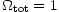at a time when few astronomers would sign on to such a high value (see [Liddle & Lyth, 1993] for a review). However, the argument for inflation goes beyond the confirmation of flatness. In particular, the discussion of the last subsection begs the question: whence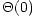, the initial conditions of the temperature fluctuations? The answer requires the inclusion of gravity and considerations of causality which point to inflation as the origin of structure in the Universe.

The calculations of the typical angular scale of the acoustic oscillations in the last section are familiar in another context: the horizon problem. Because the sound speed is near the speed of light, the degree scale also marks the extent of a causally connected region or particle horizon at recombination. For the picture in the last section to hold, the perturbations must have been laid down while the scales in question were still far outside the particle horizon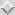. The recent observational verification of this basic peak structure presents a problem potentially more serious than the original horizon problem of approximate isotropy: the mechanism which smooths fluctuations in the Universe must also regenerate them with superhorizon sized correlations at the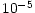level. Inflation is an idea that solves both problems simultaneously.

The inflationary paradigm postulates that an early phase of near exponential expansion of the Universe was driven by a form of energy with negative pressure. In most models, this energy is usually provided by the potential energy of a scalar field. The inflationary era brings the observable universe to a nearly smooth and spatially flat state. Nonetheless, quantum fluctuations in the scalar field are unavoidable and also carried to large physical scales by the expansion. Because an exponential expansion is self-similar in time, the fluctuations are scale-invariant, i.e. in each logarithmic interval in scale the contribution to the variance of the fluctuations is equal. Since the scalar field carries the energy density of the Universe during inflation, its fluctuations induce variations in the spatial curvature [Guth & Pi, 1985,Hawking, 1982,Bardeen et al, 1983]. Instead of perfect flatness, inflation predicts that each scale will resemble a very slightly open or closed universe. This fluctuation in the geometry of the Universe is essentially frozen in while the perturbation is outside the horizon [Bardeen, 1980].

Formally, curvature fluctuations are perturbations to the space-space piece of the metric. In a Newtonian coordinate system, or gauge, where the metric is diagonal, the spatial curvature fluctuation is called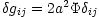(see e.g. [Ma & Bertschinger, 1995]). The more familiar Newtonian potential is the time-time fluctuation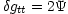and is approximately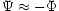. Approximate scale invariance then says that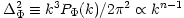where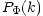is the power spectrum of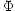and the tilt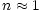.

Now let us relate the inflationary prediction of scale-invariant curvature fluctuations to the initial temperature fluctuations. Newtonian intuition based on the Poisson equation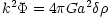tells us that on large scales (small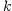) density and hence temperature fluctuations should be negligible compared with Newtonian potential. General relativity says otherwise because the Newtonian potential is also a time-time fluctuation in the metric. It corresponds to a temporal shift of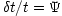. The CMB temperature varies as the inverse of the scale factor, which in turn depends on time as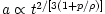. Therefore, the fractional change in the CMB temperature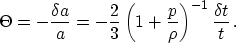(13)

Thus, a temporal shift produces a temperature perturbation of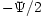in the radiation dominated era (when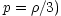and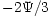in the matter dominated epoch (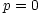) ([Peacock, 1991]; [White & Hu, 1997]). The initial temperature perturbation is therefore inextricably linked with the initial gravitational potential perturbation. Inflation predicts scale-invariant initial fluctuations in both the CMB temperature and the spatial curvature in the Newtonian gauge.

Alternate models which seek to obey the causality can generate curvature fluctuations only inside the particle horizon. Because the perturbations are then not generated at the same epoch independent of scale, there is no longer a unique relationship between the phase of the oscillators. That is, the argument of the cosine in Equation (10) becomes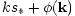, where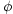is a phase which can in principle be different for different wavevectors, even those with the same magnitude. This can lead to temporal incoherence in the oscillations and hence a washing out of the acoustic peaks [Albrecht et al, 1996], most notably in cosmological defect models [Allen et al, 1997,Seljak et al, 1997]. Complete incoherence is not a strict requirement of causality since there are other ways to synch up the oscillations. For example, many isocurvature models, where the initial spatial curvature is unperturbed, are coherent since their oscillations begin with the generation of curvature fluctuations at horizon crossing [Hu & White, 1996]. Still they typically have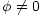(c.f. [Turok, 1996]). Independent of the angular diameter distance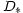, the ratio of the peak locations gives the phase: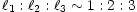for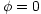. Likewise independent of a constant phase, the spacing of the peaks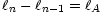gives a measure of the angular diameter distance [Hu & White, 1996]. The observations, which indicate coherent oscillations with, therefore have provided a non-trivial test of the inflationary paradigm and supplied a substantially more stringent version of the horizon problem for contenders to solve.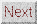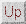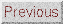Next: Gravitational Forcing Up: ACOUSTIC PEAKS Previous: Basics
Wayne Hu 2001-10-15